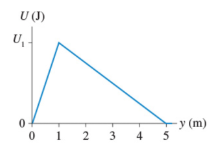# Problem: A system in which only one particle can move has the potential energy shown below. Suppose U1 = 88 JA. What is the y-component of the force on the particle at y = 0.5 m? B. What is the y-component of the force on the particle at y = 4 m?

###### FREE Expert Solution

Force:

$\overline{){\mathbf{F}}{\mathbf{=}}{\mathbf{-}}\frac{\mathbf{∆}\mathbf{U}}{\mathbf{∆}\mathbf{y}}}$

(From W = Fd cosθ and W = −ΔU)

A.

At y = 0.5m, we consider the slope of the first part of the motion between y = 0m and y = 1m.

83% (285 ratings)###### Problem Details

A system in which only one particle can move has the potential energy shown below. Suppose U= 88 JA. What is the y-component of the force on the particle at y = 0.5 m?

B. What is the y-component of the force on the particle at y = 4 m?